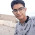## TRENDING

### "R.D SHARMA OBJECTIVE [PART-1]"

ALGEBRA

1. ALGEBRAIC INEQUATIONS
2. LOGARITHMS
3. MISCELLANEOUS EQUATIONS AND INEQUATIONS
4. INEQUALITIES
5. SETS
6. CARTESIAN PRODUCT OF SETS AND RELATIONS
7. FUNCTIONS
8. MATHEMATICAL REASONING
9. COMPLEX NUMBERS
10. SEQUENCES AND SERIES
12. MATHEMATICAL INDUCTION
13. PERMUTATION AND COMBINATIONS
14. BINOMIAL THEOREM AND ITS EXPRESSION, APPLICATIONS
15. EXPONENTIAL AND LOGARITHMIC SERIES
16. MATRICES
17. DETERMINANTS
18. PROBABILITY
19. DISCRETE PROBABILITY DISTRIBUTION

### "R.D SHARMA OBJECTIVE [PART-2]"

TRIGONOMETRY

27. TRIGONOMETRIC RATIOS AND IDENTITIES
28. PROPERTIES OF TRIANGLES AND CIRCLES CONNECTED WITH THEM
29. SOLUTIONS OF TRIANGLES
30. INVERSE TRIGONOMETRIC FUNCTIONS
31. TRIGONOMETRIC EQUATIONS AND INEQUATIONS
32. HEIGHT AND DISTANCE

CALCULUS

33. REAL FUNCTIONS
34. LIMITS
35. CONTINUITY AND DIFFERENTIABILITY
36. DIFFERENTIATION
37. TANGENTS AND NORMALS
38. DERIVATIVE AS A RATE MEASURER
39. DIFFERENTIALS,ERRORS AND APPROXIMATIONS
40. MEAN VALUE THEOREMS
41. MAXIMA AND MINIMA

42. INCREASING AND DECREASING FUNCTIONS
43. INDEFINITE INTEGRALS
44. DEFINITE INTEGRALS
45. AREAS OF BOUNDED REGION
46. DIFFERENTIAL EQUATIONS

VECTORS AND 3-DIMENSIONAL GEOMETRY

47. ALGEBRA OF VECTORS
48. SCALER AND VECTOR PRODUCTS OF TWO VECTORS
49. SCALER AND VECTOR PRODUCTS OF THREE VECTORS
50. THREE DIMENSIONAL COORDINATE SYSTEM
51. PLANE AND STRAIGHT LINE IN SPACE
52. MEASURES OF CENTRAL TENDENCY
53. MEASURES OF DISPERSION

# This Website helps student who can’t buy costly books. This Website provide Kota institute material’s, latest book by iitians, fiitjee material, handbook of chemistry, ALLEN DLP etc.  This website is useful if you are preparing for JEE main entrance exam, IIT entrance exam, JEE (main + advanced)

### ☆ JUST WANT TO SAY, PLEASE SHARE THE BLOG AS MUCH AS POSSIBLE, SO THAT IT COULD HELP OTHER MILLION OF IIT-JEE/NEET ASPIRANTS.....

1.hi sir
i am also reachable at ibwithiyer@gmail.com

1.2.